# Banach-Steinhaus theorem

(diff) ← Older revision | Latest revision (diff) | Newer revision → (diff)
A general appellation for several results concerning the linear-topological properties of the space of continuous linear mappings of one linear topological space into another. Letandbe locally convex linear topological spaces, where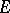is a barrelled space, or letand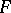be linear topological spaces, whereis a Baire space. The following propositions are then valid. 1) Any subset of the setof continuous linear mappings ofintowhich is bounded in the topology of simple convergence is equicontinuous (the uniform boundedness principle); 2) If a filterin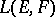contains a set bounded in the topology of simple convergence, and converges in the topology of simple convergence to some mapping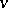ofinto, thenis a continuous linear mapping ofinto, and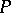converges uniformly toon each compact subset of, .
These general results make it possible to render the classical results of S. Banach and H. Steinhaus  more precise: Letandbe Banach spaces and letbe a subset of the second category in. Then, 1) ifand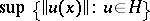is finite for all, then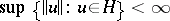; 2) if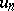is a sequence of continuous linear mappings ofinto, and if the sequenceconverges infor all, thenconverges uniformly on any compact subset ofto a continuous linear mappingofinto.